KINETIC ENERGY. Due to its position it also contains POTENTIAL ENERGY. The total energy contained by an electron (kinetic plus potential) is the factor which determines the radius of the electron orbit."> Energy LevelsCustom Search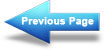Matter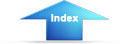Matter, Energy, and ElectricityValenceEnergy Levels

Since an electron in an atom has both mass and motion, it contains two types of energy. By virtue of its motion the electron contains KINETIC ENERGY. Due to its position it also contains POTENTIAL ENERGY. The total energy contained by an electron (kinetic plus potential) is the factor which determines the radius of the electron orbit. In order for an electron to remain in this orbit, it must neither GAIN nor LOSE energy.

It is well known that light is a form of energy, but the physical form in which this energy exists is not known.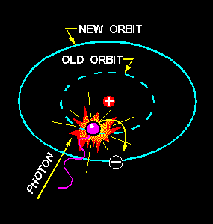Once the electron has been elevated to an energy level higher than the lowest possible energy level, the atom is said to be in an excited state. The electron will not remain in this excited condition for more than a fraction of a second before it will radiate the excess energy and return to a lower energy orbit. To illustrate this principle, assume that a normal electron has just received a photon of energy sufficient to raise it from the first to the third energy level. In a short period of time the electron may jump back to the first level emitting a new photon identical to the one it received.

A second alternative would be for the electron to return to the lower level in two jumps; from the third to the second, and then from the second to the first. In this case the electron would emit two photons, one for each jump. Each of these photons would have less energy than the original photon which excited the electron.

This principle is used in the fluorescent light where ultraviolet light photons, which are not visible to the human eye, bombard a phosphor coating on the inside of a glass tube. The phosphor electrons, in returning to their normal orbits, emit photons of light that are visible. By using the proper chemicals for the phosphor coating, any color of light may be obtained, including white. This same principle is also used in lighting up the screen of a television picture tube.

The basic principles just developed apply equally well to the atoms of more complex elements. In atoms containing two or more electrons, the electrons interact with each other and the exact path of any one electron is very difficult to predict. However, each electron lies in a specific energy band and the orbits will be considered as an average of the electron's position.

Q7.What is energy of motion called?   Test Yourself
Q8.How is invisible light changed to visible light in a fluorescent light?   Test Yourself

Shells and Subshells

The difference between the atoms, insofar as their chemical activity and stability are concerned, is dependent upon the number and position of the electrons included within the atom. How are these electrons positioned within the atom? In general, the electrons reside in groups of orbits called shells. These shells are elliptically shaped and are assumed to be located at fixed intervals. Thus, the shells are arranged in steps that correspond to fixed energy levels. The shells, and the number of electrons required to fill them, may be predicted by the employment of Pauli's exclusion principle. Simply stated, this principle specifies that each shell will contain a maximum of 2n2electrons, where n corresponds to the shell number starting with the one closest to the nucleus. By this principle, the second shell, for example, would contain 2(2)2 or 8 electrons when full.

In addition to being numbered, the shells are also given letter designations, as pictured in figure 1-3. Starting with the shell closest to the nucleus and progressing outward, the shells are labeled K, L, M, N, O, P, and Q, respectively. The shells are considered to be full, or complete, when they contain the following quantities of electrons: two in the K shell, eight in the L shell, 18 in the M shell, and so on, in accordance with the exclusion principle. Each of these shells is a major shell and can be divided into subshells, of which there are four, labeled s, p, d, and f. Like the major shells, the subshells are also limited as to the number of electrons which they can contain. Thus, the "s" subshell is complete when it contains two electrons, the "p" subshell when it contains 10, and the "f" subshell when it contains 14 fourteen electrons.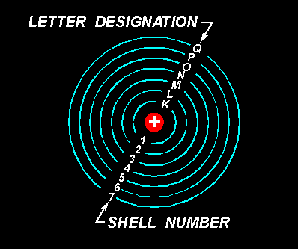In as much as the K shell can contain no more than two electrons, it must have only one subshell, the s subshell. The M shell is composed of three subshells: s, p, and d. If the electrons in the s, p, and d subshells are added, their total is found to be 18, the exact number required to fill the M shell. Notice the electron configuration for copper illustrated in figure 1-4. The copper atom contains 29 electrons, which completely fill the first three shells and subshells, leaving one electron in the "s" subshell of the N shell.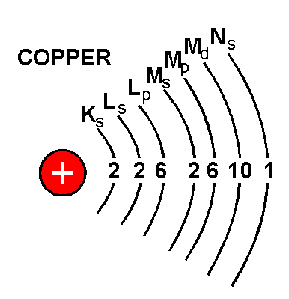Integrated Publishing, Inc. - A (SDVOSB) Service Disabled Veteran Owned Small Business